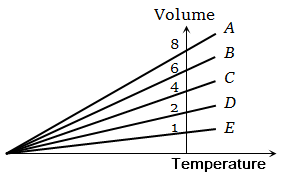The expansion of an ideal gas of mass m at a constant pressure P is given by the straight line D. Then the expansion of the same ideal gas of mass 2m at a pressure P/ 2 is given by the straight line , where number on graphs indicate slope , is-1.  E

2.  C

3.  B

4.  A